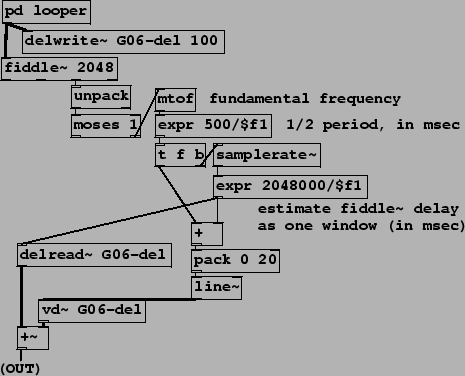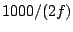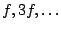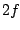Next: Time-varying complex comb filter: Up: Examples Previous: Order of execution in   Contents   Index

Non-recirculating comb filter as octave doubler

In example G06.octave.doubler.pd (Figure 7.29) we revisit the idea of pitch-based octave shifting introduced earlier in E03.octave.divider.pd. There, knowing the periodicity of an incoming sound allowed us to tune a ring modulator to introduce subharmonics. Here we realize the octave doubler described in Section 7.3. Using a variable, non-recirculating comb filter we take out odd harmonics, leaving only the even ones, which sound an octave higher. As before, the spectral envelope of the sound is roughly preserved by the operation, so we can avoid the chipmunk" effect we would have got by using speed change to do the transposition.The comb filtering is done by combining two delayed copies of the incoming signal (from the pd looper subpatch at top). The fixed one (delread~) is set to the window size of the pitch following algorithm. Whereas in the earlier example this was hidden in another subpatch, we can now show this explicitly. The delay in milliseconds is estimated as equal to the 2048-sample analysis window used by the fiddle~ object; in milliseconds this comes towhereis the sample rate.

The variable delay is the same, plus 1/2 of the measured period of the incoming sound, ormilliseconds whereis the frequency in cycles per second. The sum of this and the fixed delay time is then smoothed using a line~ object to make the input signal for the variable delay line.

Since the difference between the two delays is, the resonant frequencies of the resulting comb filter are; the frequency response (Section 7.3) is zero at the frequencies, so the resulting sound contains only the partials at multiples of--an octave above the original. Seen another way, the incoming sound is output twice, a half-cycle apart; odd harmonics are thereby shifted 180 degrees (radians) and cancel; even harmonics are in phase with their delayed copies and remain in the sum.

Both this and the octave divider may be altered to make shifts of 3 or 4 to one in frequency, and they may also be combined to make compound shifts such as a musical fifth (a ratio of 3:2) by shifting down an octave and then back up a factor of three. (You should do the down-shifting before the up-shifting for best results.)Next: Time-varying complex comb filter: Up: Examples Previous: Order of execution in   Contents   Index
Miller Puckette 2006-12-30